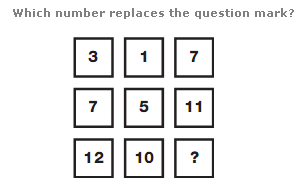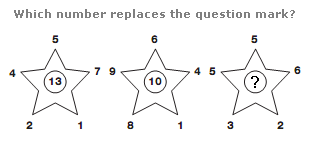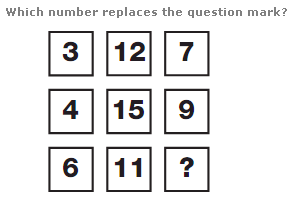# Puzzles - Number puzzles

### Exercise :: Number puzzlesAnswer : 16 Explanation : Working in columns, starting with the top number, add 4 to give the next value down, and add 5 to give the one under that.Answer : 11 Explanation : In each star, add together the top 3 numbers, and subtract the lower 2 numbers, to give the value in the centre of each star.Answer : 3 Explanation : In each row of the diagram, add the left and right hand digits together and add 2 to give the result in the centre.